Function Repository Resource:

# TurningNumber

Compute the turning number of a curve

Contributed by: Wolfram Staff (original content by Alfred Gray)
 ResourceFunction["TurningNumber"][c,{t,a,b}] computes the turning number of a curve c with parameter t running from a to b.

## Details and Options

ResourceFunction["TurningNumber"] is similar to winding number, but in this case the number of rotations is counted with respect to the tangent vector of the curve instead of a fixed point.

## Examples

### Basic Examples (1)

The turning number changes its sign depending on whether the tangent vector moves clockwise or counterclockwise:

 In:=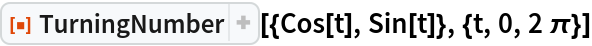Out=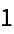In:=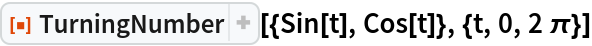Out=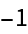### Scope (2)

Define a limaçon:

 In:=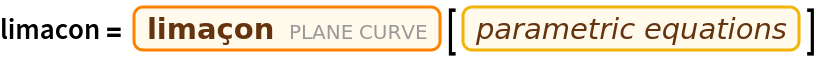Out=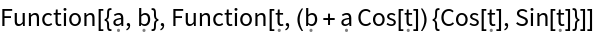Here is a function for computing tangent vectors:

 In:=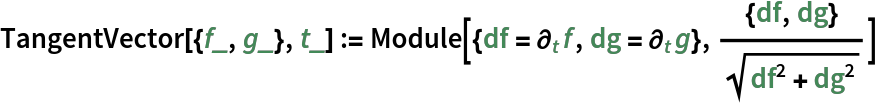Interactively plot the limaçon while tracking a tangent vector and its turning number. This shows that the turning number of a limaçon is 2:

 In:=Out=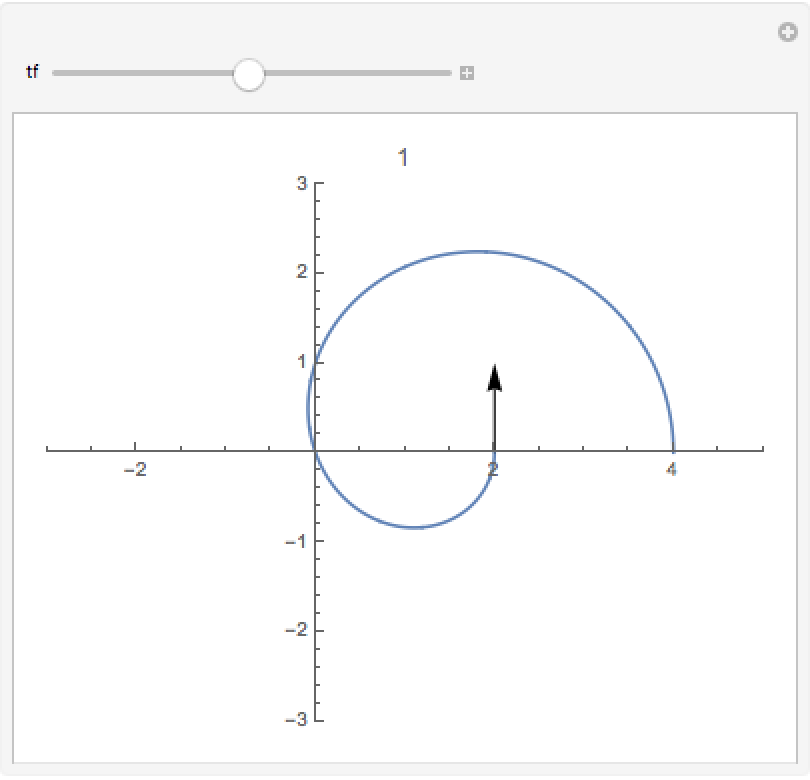Define an eight curve:

 In:=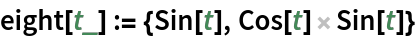The turning number of an eight curve is first -1, then 1, giving a total of 0:

 In:=Out=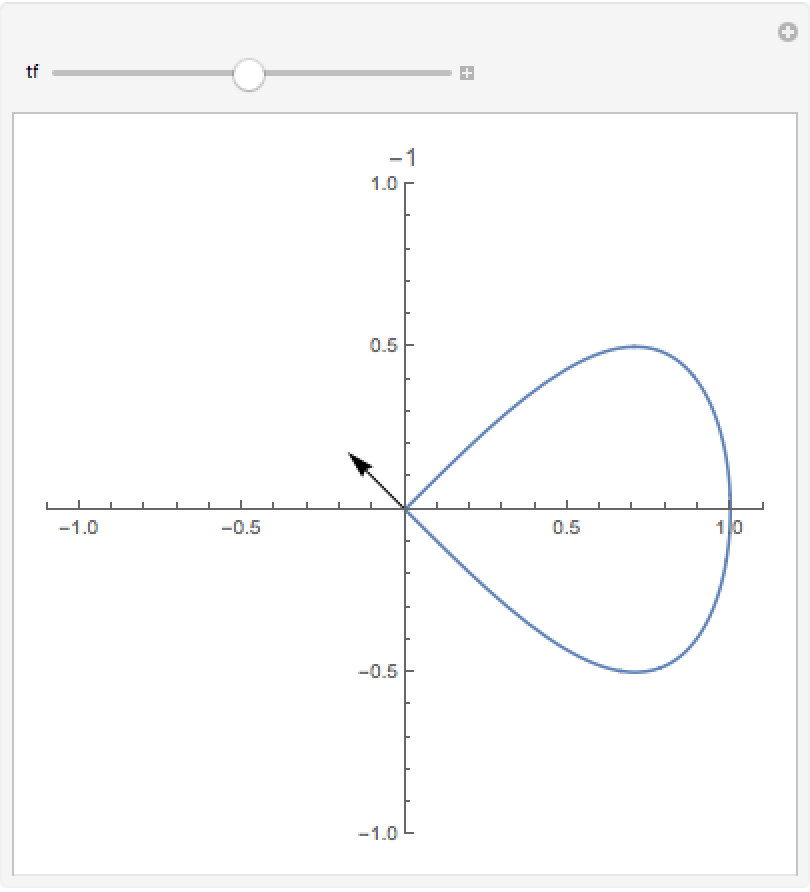Enrique Zeleny

## Version History

• 1.0.0 – 21 February 2020